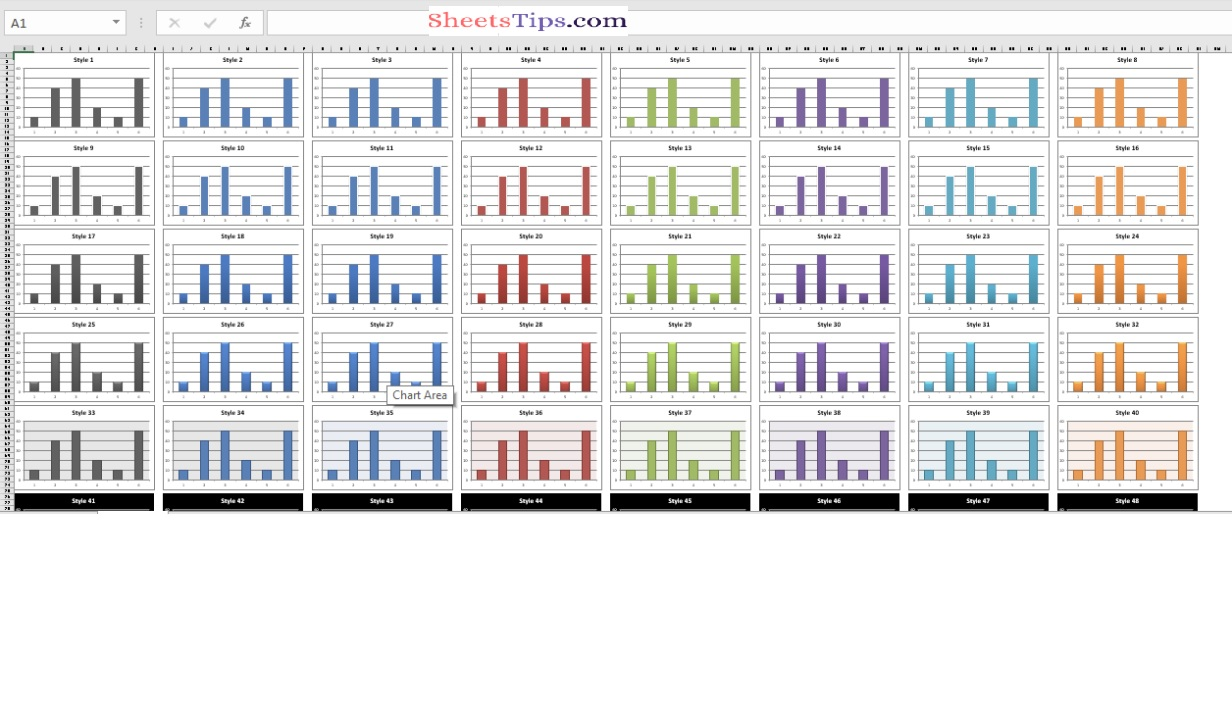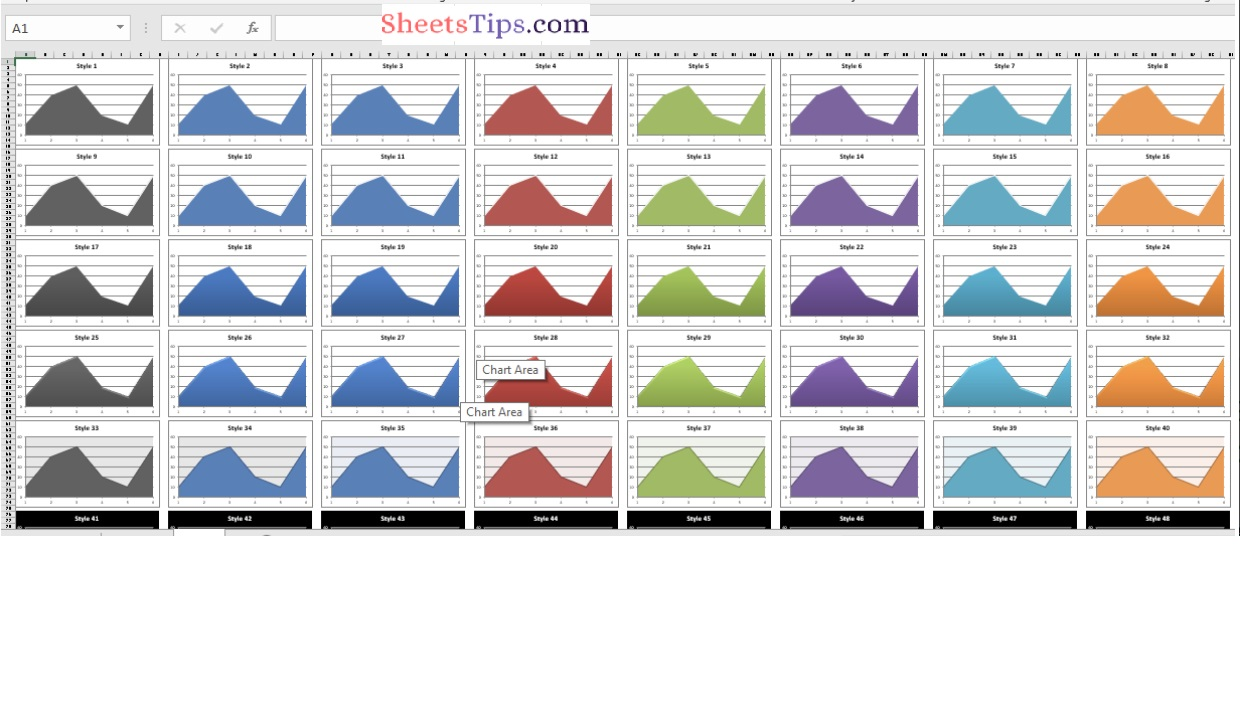# Python Program to Plot Different types of Style Charts in Excel Sheet using XlsxWriter module

Below are the ways to plot the different types of style charts in an excel sheet using the XlsxWriter module in Python.

Charts are made up of a series of one or more data points. The series are made up of references to cell ranges. To plot the charts on an excel sheet, first create a chart object of the desired chart type ( i.e Stock chart, etc.). After generating chart objects, insert data into them before adding them to the sheet object.

Install the xlsxwriter using the below code

`pip install xlsxwriter`

Looking in indexes: https://pypi.org/simple, https://us-python.pkg.dev/colab-wheels/public/simple/
Collecting xlsxwriter
|████████████████████████████████| 149 kB 5.0 MB/s
Installing collected packages: xlsxwriter
Successfully installed xlsxwriter-3.0.3

## Program to Plot Different types of Style Charts in Excel Sheet using XlsxWriter module in Python

Approach:

• Import the xlsxwriter library using the import function.
• Pass the excel file path to the Workbook() function of xlsxwriter which is the file we want to create and store in a variable.
• Give the chart types as a static list and store them in a variable.
• Iterate in the chart types list using the for loop.
• Create the new Worksheet using the add_worsheet() function and apply it to the above workbook object and store it in a variable.
• Pass the Argument chartType(Iterator) and apply title() function to it.
• Set the zoom option using the set_zoom() function and pass the zoom value to it as an argument.
• Take a variable and initialize the StyleNumber with some random value.
• Creating the 48 Built-in styles where each style is a different style.
• Here the each chart dimension is 15 X 8.
• Using the add_chart() method, create a chart object that can be added to a worksheet.
• Pass the type of chart as an argument to the add_chart() function and apply it to the above workbook object.
• Set the title to the chartObject using the set_title() function by passing the title styleNumber as value and key as “name” (Here the arguments are object).
• Turn off the chart legend by passing the key ‘none’ as True to the set_legend() function.
• Set the style of the chart using the set_style() function.
• Add the chart Object to the worksheet by passing the row Number, Column, and chart object as argument to the insert_chart() function.
• Increment the style Number by 1.
• Create the new Worksheet using the add_worsheet() function and apply it to the above workbook object and store it in a variable.
• Here this worksheet is used to store the data.
• Create some random data list and store it in a variable.
• Write the dataValues to the A1 column of the data Worksheet using the write_column() function and apply on the data Worksheet.
• Hide the data Worksheet using the hide() function.
• Close the workbook Object using the close() function.
• The Exit of the Program.

Below is the Implementation:

```# Import the xlsxwriter library using the import function
import xlsxwriter

# Pass the excel file path to the Workbook() function of xlsxwriter
# which is the file we want to create and store it in a variable
workbookObj = xlsxwriter.Workbook('chartStyles.xlsx')

# Give the chart types as static list and store it in a variable
chartTypes = ['column', 'area']

# Iterate in the chart types list using the for loop
for chartType in chartTypes:

# Create the new Worksheet using the add_worsheet() function and
# apply it to the above workbook object and store it in a variable
#Pass the Argument chartType(Iterator) and apply title() function to it.

# set the zoom option using the set_zoom() function and pass the zoom value to it as an argument
newWorksheet.set_zoom(20)

# Take a variable and initialize the StyleNumber with some random value
styleNumber = 1

# Creating the 48 Built in styles where each style is different style
# Here the each chart dimension is 15 X 8
for rowNum in range(0, 90, 15):
for colNum in range(0, 64, 8):

# Using the add_chart() method, create a chart object that can be added to a worksheet.
# Pass the type of chart as an argument to the add_chart() function and apply it to the above workbook object

# Set the title to the chartObject using the set_title() function by passing the title styleNumber as value and key as "name" (Here the arguments are object)
chartObj.set_title ({'name': 'Style % d' % styleNumber})

# Turn off the chart legend by passing the key 'none' as True to the set_legend() function
chartObj.set_legend({'none': True})

# Set the style of the chart using the set_style() function
chartObj.set_style(styleNumber)

# Add the chart Object to the worksheet by passing the
# row Number,Column and chartObject as argument to the insert_chart() function
newWorksheet.insert_chart(rowNum, colNum, chartObj)

# Increment the style Number by 1
styleNumber += 1

# Create the new Worksheet using the add_worsheet() function and
# apply it to the above workbook object and store it in a variable
# Here this worksheet is used to store the data

# Create some random data list and store it in a variable
dataValues = [20, 30, 40, 10, 20, 60]

# Write the dataValues to the A1 column of the data Worksheet using the write_column() function and apply on the data Worksheet

dataWorksheet.write_column('A1', dataValues)

# Hide the data Worksheet using the hide() function
dataWorksheet.hide()

# Close the workbook Object using the close() function.
workbookObj.close()

```

Output: